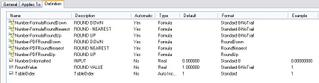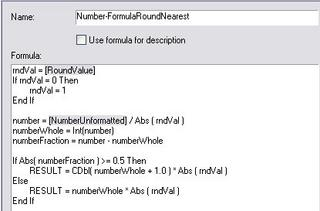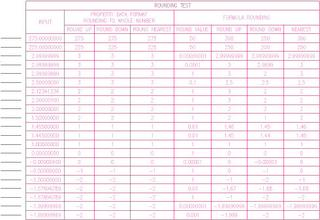## September 03, 2005

### Rounding Redux

UPDATE, 9/10/2005: Jon Smith of Construction Industry Solutions (COINS) has pointed out that the problem with the rounding using Property Data Formats in my sample file was that I had the Round Value set to "0"; the problem disappears if you use "1" instead. I have confirmed that this is true for ADT 2006 and ADT 2005. I will post back with results in ADT 2004 when I get a chance to try my new test file at work next week. In the mean time, the new test file is available from the same thread in the Autodesk Architectural Desktop Content Discussion Group to which I posted the previous test file.

In order to provide flexibility and to match the rounding functionality present in Property Data Formats, I added a new, real number manual property, RoundValue. This provides the equivalent of the Round Off Value in the Property Data Formats. The default value is 1, which rounds to the nearest whole number. To round to two decimal places, enter 0.01. To round to one half, enter 0.5. To round to the nearest 100, enter 100. The math behind the function involves division, so entering 0 would cause an undefined value. The formulas convert an entered value of 0 to 1.

There are three new formulas - one to round up, one to round down and one to round to nearest. I considered setting up nearest to work like the VBScript function, where exactly 5 in the digit beyond the rounding digit would round up for odd numbers in the rounding digit and down for even numbers in the rounding digit. While that may make some sense when the RoundValue is 1 times 10 to some power, for RoundValues like 50, where 275 would round up to 300 but 225 would round down to 200, the "oddness" or "eveness" of the starting number was not readily apparent. I chose to take the easy way out and simply have exactly 5 always round up. The properties in my revised LineObjects Property Set Definition are shown below.LineObjects Property Set Definition

The new formulas also work with negative numbers, should your application require that. I chose to treat negative numbers such that rounding up goes to a higher number and down goes to a smaller number. For example, -10.5 would be rounded up to -10 and down to -11. I changed the function I used for rounding from Fix, used in the previous rounding formulas, to Int, as that handles negative numbers in the way previously described. [For negative numbers, Fix rounds up away from zero and down towards zero; in other words, it rounds up to the greater absolute value. Fix and Int return the same results for positive numbers.]

The Number-FormulaRoundDown formula property is the simplest. After converting any zero value in RoundValue to 1, the formula divides the source number by the absolute value of RoundValue, uses the Int function to truncate any decimal portion, then multiplies that whole number portion by the absolute value of RoundValue to arrive at the desired result. The formula, as it appears in ADT 2006, is shown below.Number-FormulaRoundDown Formula

The Number-FormulaRoundUp formula property works in a similar fashion, dividing by the RoundValue, truncating any fraction and multiplying that by the RoundValue, but needs to determine if there is any fractional part that would require rounding up. This is done by storing intermediate results in variables, calculating the fractional part and, if any, adding one to the whole number before finally multiplying it by the absolute value of RoundValue to get the final result. The formula is shown below.Number-FormulaRoundUp Formula

The Number-FormulaRoundNearest formula property is almost identical to the round up property, except that adding one to the whole number value only happens if the fractional part is 0.5 or greater. The formula is shown below.Number-FormulaRoundNearest Formula

There is a schedule table in the sample file that shows the results of various sample values and, for the formulas, various RoundValues. As before, lines are used as the objects to hold the properties. As previously noted the table is sorted by the vaule in TableOrder; the TableOrder column is hidden in the Schedule Table Style. Feel free to play around with the values assigned to any line to see how the results of the different rounding formulas change.Rounding Test Schedule Table

You should be able to adapt one of these formulas to accomplish any property data rounding needs you may have, until such time as the rounding feature in the Property Data Formats is corrected. When you substitute the property you wish to round for the NumberUnformatted property in the sample file, make certain that you have a number and not a string. Read more about creating unformatted numeric properties in my previous blog article on that subject. Also note that if, for a given formula, the rounding value will always be the same, you do not need to have the RoundValue property, and can enter the number directly in the formula, by replacing each instance of "Abs ( rndVal )" with your desired value [use a positive value]. In that case, you can delete the first five lines, which read in the value of RoundValue and substitute 1 if the value is 0.